- BetterExplained - https://betterexplained.com -

# Intuition For Graphed Functions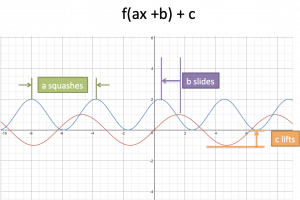When we graph a function $f(x)$, there's a few ways we can modify it: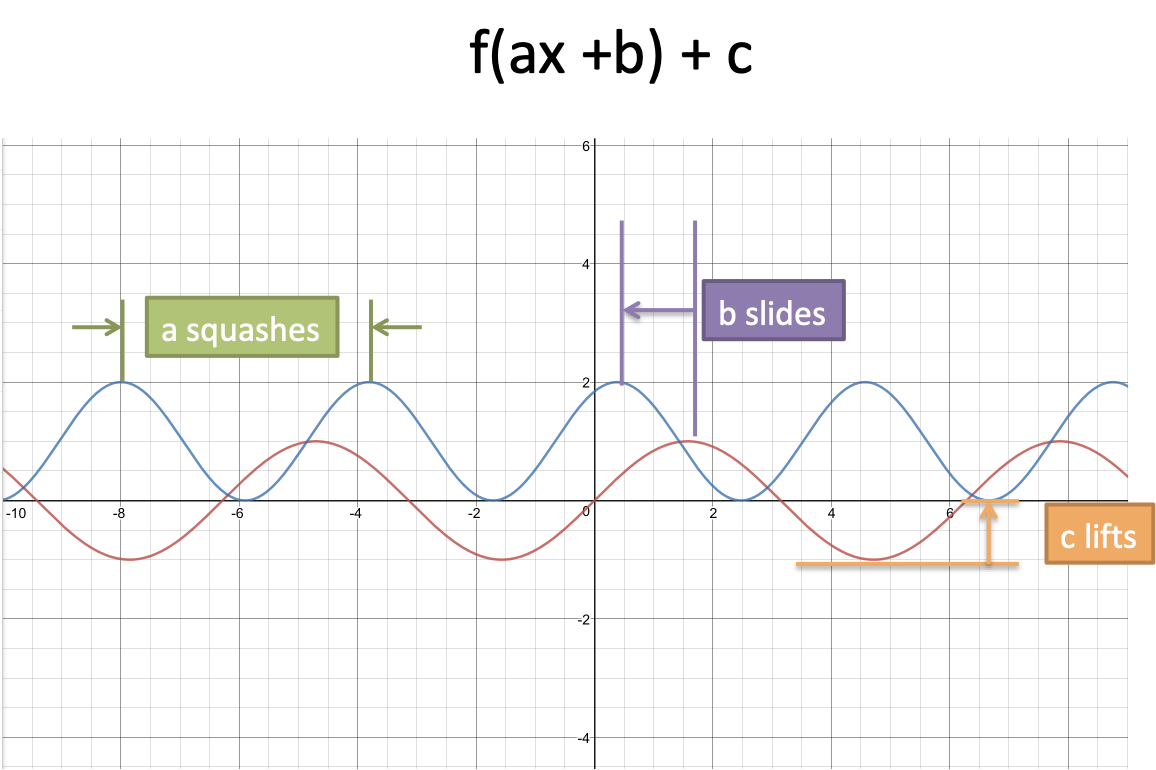Using $f(ax + b) + c$ instead of $f(x)$ has a few effects:

What's going on? Well, we're describing the visual result on the graph, but aren't describing that underlying process that made the change. Let's take a look at the root cause.

## f(ax) is fast forward

The "a" in f(ax) is a fast-forward factor. Normally, we experience time as "1 minute per minute" -- for every minute we wait, the world advances one minute into the future.

What if we saw life on fast forward?

Here, 1 minute passing to us (in our "x" timeline) means 2 minutes passed in the real world. Or 10 minutes, an hour, or a year.

On our timeline (x), time passes as normal. But our function, which determines the results we see, is being fed a modified timeline. While we leisurely stroll from x=1 to x=2, f has to jump from f(1) to f(2) to f(3) ... up to f(20). Here, f needs to graph 10 minutes of events while we casually waited one minute. Cramming more data points into the same time period is a squashed, sped-up graph.

Intuitively, "Squashing the graph" really means "running time faster".

## f(x + b) is an offset

A simpler version of time travel is a basic shift. If things happen ahead of schedule, what does that mean?

Imagine a German/Japanese city where the trains run an hour ahead of schedule.

The 4pm train arrives at 3pm. The 11am flight takes off at 10am. In other words, a dystopian hellscape.

If I managed not to burst into flames upon arriving, I'd describe the situation like this:

• Actual time: 10am
• Flight that leaves: 11am
• Flight = actual + 1

In other words, f(x + 1) means things run ahead of schedule. We think it's 3pm (x = 3), but the 4pm events are happening [f(4) is happening].

Again, this can be tricky: doesn't it seem like we remove time to make things happen earlier? This is our visual intuition fooling us: if it's 3pm but f is running the 4pm events, it's going ahead of schedule. Note that we add time to our watch in order to arrive early.)

See "slide to the left" as "ahead of schedule".

## f(x) + c is a bias

Adding a value to a function moves it vertically.

What's happening? Unbeknownst to f(x), we take take the final result and make it larger.

Intuitively, we have a "bias". When f(x) = 0, it's telling us "don't change, stay at 0". Except our default value isn't zero, it's c. When f(x) says don't change, that means "use your default value, c".

See "sliding up the function" as "changing the default value".

(In neural networks, you might have a default value if there's zero input. A "default bias" is a nice way to describe this, vs. "vertically sliding the function".)

## Appendix: Chain Rule

In Calculus, the chain rule lets us compose functions. (Fancy phrase for cramming one function inside another.)

When we cram 2x inside of sin, and take the derivative, we get: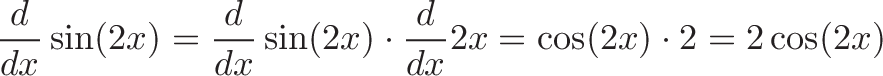$\displaystyle{\frac{d}{dx}\sin(2x) = \frac{d}{dx}\sin(2x) \cdot \frac{d}{dx} 2x = \cos(2x) \cdot 2 = 2 \cos(2x)}$

The chain rule tells us to take the derivative of the outer function (sin(2x) => cos(2x)) and multiply by the derivative of the inner function (2x => 2).

What's going on? Using the "derivative = slope" interpretation (not my favorite but good for graphing), we see this: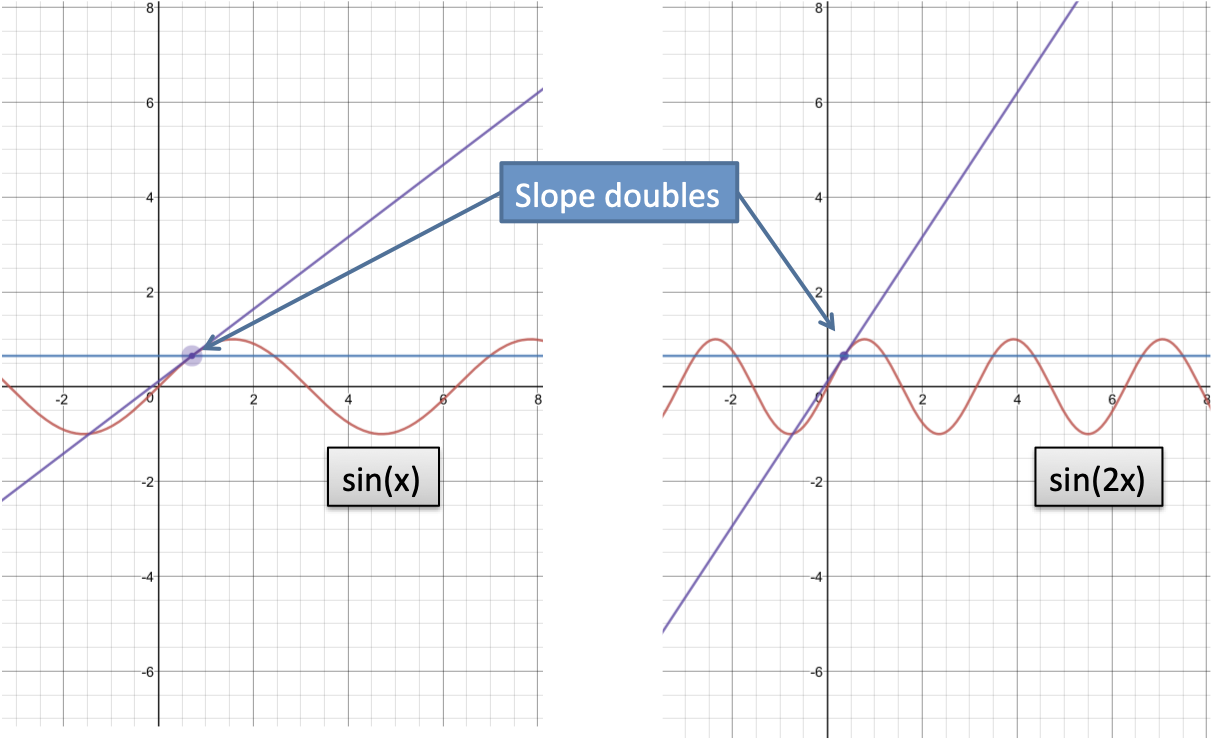If we pick a point on the cycle (such as x = 1 radian), we find the slope there as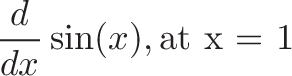$\displaystyle{\frac{d}{dx} \sin(x), \text{at x = 1}}$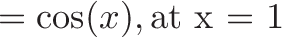$\displaystyle{ = \cos(x), \text{at x = 1} }$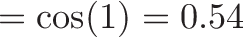$\displaystyle{ = \cos(1) = 0.54 }$

In other words, at x = 1, sin(x) has a nice upward slope of about 54%. Ok, great.

Now, what happens if we run sin(x) at twice the speed? Eat, eat, eat!

Well... the derivative (slope we see) should double! Compared to the original, sin(2x) runs through changes twice as fast as we do. 1 minute in our world means sin(2x) has chomped through 2 minutes of changes.

At the corresponding point in the cycle, we should expect double the slope. Let's make sure.

Instead of asking for x = 1, we know that same point on sin(2x)'s timeline is now x = 0.5. So let's ask for the derivative there: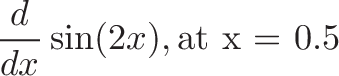$\displaystyle{\frac{d}{dx} \sin(2x), \text{at x = 0.5}}$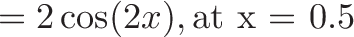$\displaystyle{ = 2\cos(2x), \text{at x = 0.5} }$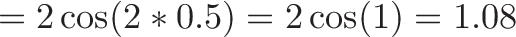$\displaystyle{ = 2\cos(2 * 0.5) = 2 \cos(1) = 1.08}$

Yay, the math worked!

We can mechanically describe the chain rule as a way to compose functions. But intuitively, we've strapped a fast-forward device inside our function, speeding up the changes we experience.

Regular sin(x) keeps its derivative between -1.0 and +1.0, the limits imposed by cos(x). But now we have a fast-forward trick to make sin(x) move as fast as we want.

Extra: Imagine we weren't fast-forwarding at a constant rate of 2; what if sped up more, the further along we went? That function could be sin(x * x), where one x is our regular location in the cycle, and the other x is our fast-forward rate. For fun (yes!), you can take the derivative of sin(x * x) (answer).

## Appendix: Exponential Functions

We can run the exponential function ($e^x$) ahead of schedule with $e^{x + b}$.

But we can rewrite this to: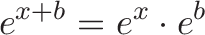$\displaystyle{e^{x + b} = e^x \cdot e^b}$

In other words, running the exponential function "ahead of schedule" can be seen as the regular exponential function with a bigger starting point.

Normal exponentials start at 1.0 and begin compounding continuously. Instead, we can see it as starting with a bigger starting value from 1.0. (For example, $e^{x + 2}$ starts compounding from $e^2 = 7.389$.)

Depending on the function, interpretations other than "ahead of schedule" might make more sense.

Happy math.